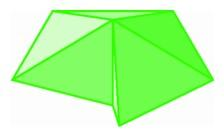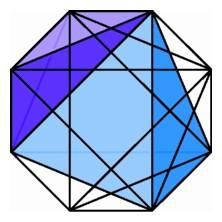The Hypercube Dual or Sixteen-CellFour tetrahedra around an edge in three-space.

The dihedral angle of a tetrahedron is less than a right angle, so we can easily fit four tetrahedra around an edge in three-space, with room to fold up into four-space. There is a regular polytope that has this configuration of four tetrahedra at each edge, and to describe its construction we rely on the duality principle. To construct the dual of a polytope, we choose the vertex in the center of each three-dimensional face, and we connect vertices from faces that share a common boundary polygon. The vertices coming from three-dimensional faces sharing a vertex determine a three-dimensional polyhedron, the dual cell of the vertex. The collection of dual cells forms the dual polytope of the regular polytope.

Like the three-simplex or tetrahedron, the four-simplex is self-dual. To describe the dual of the hypercube, we choose a point in the center of each of the eight cubical faces. Around each of the 16 vertices there are four cubes, each set of four contributing a tetrahedron to the dual object. We thus obtain a new regular polytope, the 16-cell, dual to the hypercube, and analogous to the octahedron, which is dual to the cube in three-space. This polytope has four tetrahedra around each edge, and it is the third of the regular polytopes in four-space.Projection of the 16-cell into the plane, showing 2 of the 16 three-simplexes. The others are obtained by rotating the figure by multiples of 45 degrees.